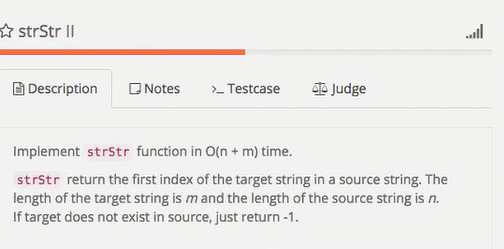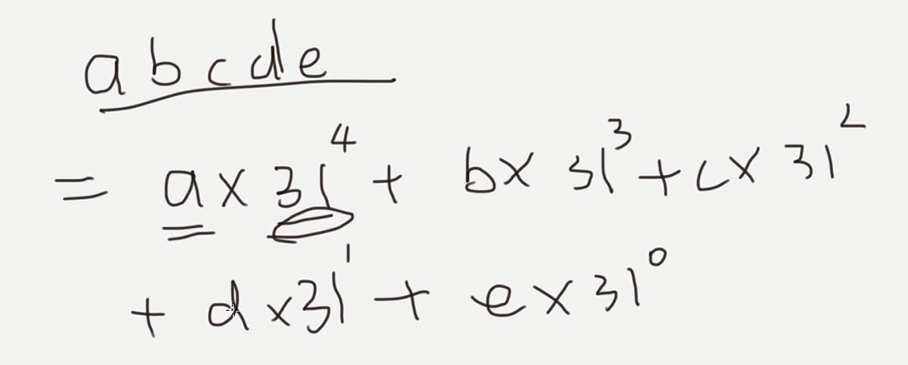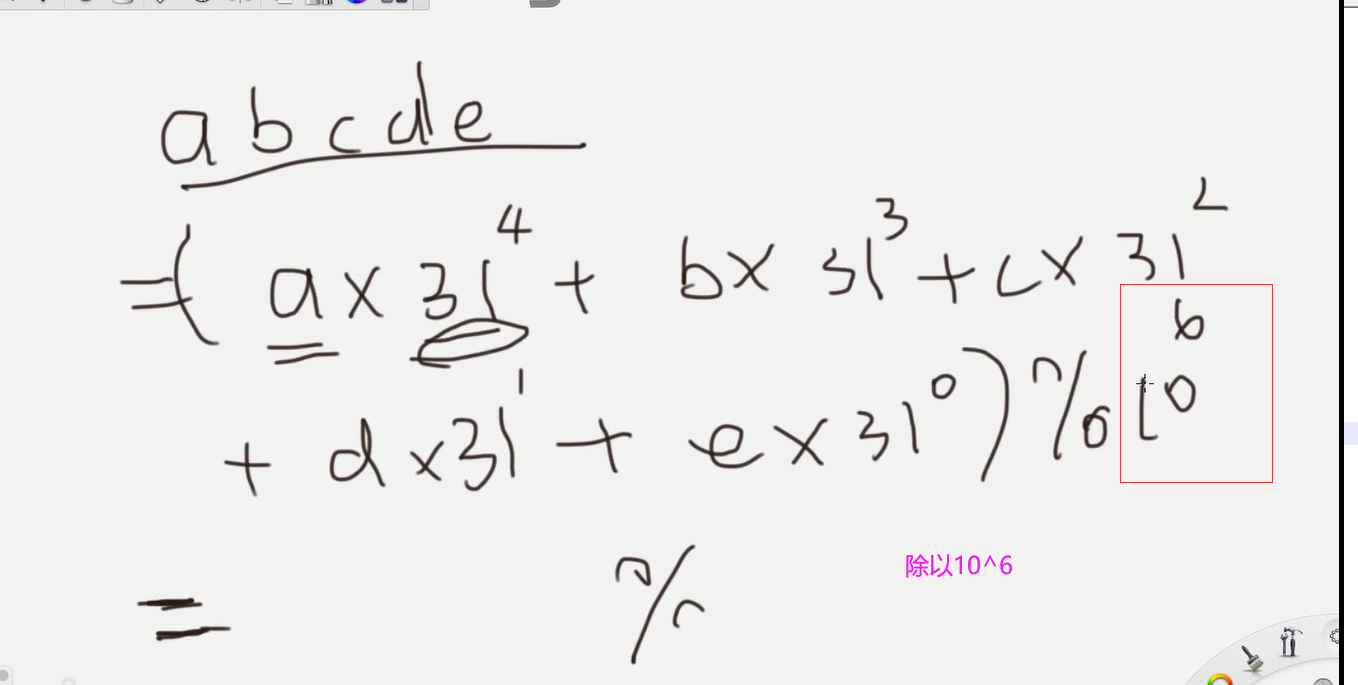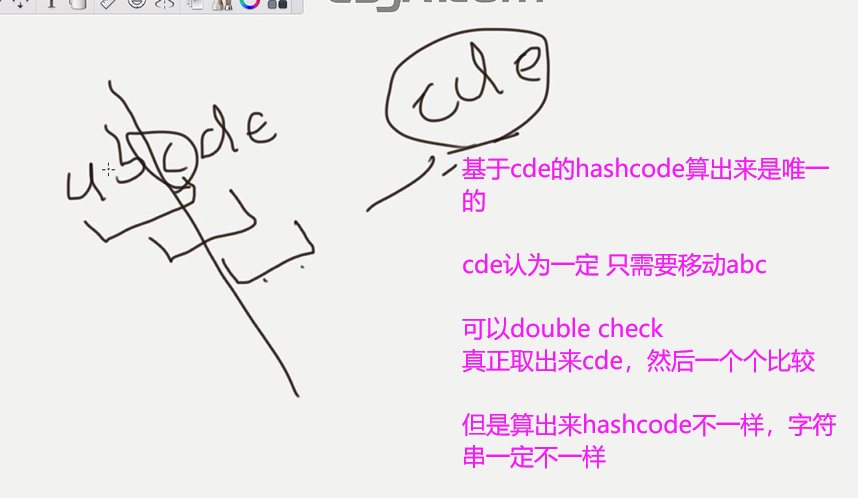# 基础班 strStr算法实现hash function 把一个string对应到某一个位置 ，然后下次他来的时候还是这个位置，可以检查看是否存在于整个数组当中Rabin Karp Algorithm。用Hash方程的思想做。自己实现Hash function。
1.提前算好target的code。
2.开始读入source的每个字符，在for循环里计算所有长度为target.length()的子字符串的hashcode。
3.对比，如果code一样，要进一步对比是否substring的确一样以排除false positive.

1.第二步内比如从abc -> bcd，for循环每次都加了新的字符，d已经被加上去了，你只要轻松地排除窗口刚滑走的a幂次即可。因为幂次固定是31target.length()，可以提早把幂次算好。
2.对%BASE这个操作每次计算别忘了。
3.对两个各自对BASE取余过的数做减法后，可能会出来负数，这时候不好

public class Solution{
public int BASE=1000000
public int strStr2(String source,String target){
if(source==null||target==null){
return -1;
}
//再检查是否是空串 空串可以认为是字符串的第0个位置开始
int m=target.length();//把length取出来
if(m==0){
return 0;
}

//1^m  一边乘一边模可以保证不越界 // 31^length, 31 is HASHBASE.
//这个power是用来在sliding window时，去掉左边的character的hash的
int power=1;
for(int i=0;i<m;i++){
power=(power*31)%BASE
}
//这个i我也是很迷 循环了power

int targetCode=0;
for (int i=0;i<m;i++){
targetCode=(targetCode*31+target.charAt(i))%BASE;
}//乘以31+当前的  想象等比数列

int hashCode=0;

for (int i=0;i<source.length();i++){
//abc+d
hashCode=(hashCode*31+source.charAt(i))%BASE;
//如果不够m个字符，没必要做后面的呢操作
if(i<m-1){
continue;
}

//abcd-a  abc-->bcd
if(i>=m){
hashCode=hashCode-(source.char(i-m)*power)%BASE;
//hashCode=(hashCode-(source.char(i-m)*power)%BASE)%BASE;
//括号注意，直接晕了  base
//不要这样，因为不同的语言，负数，出来的结果不一样
//两个数都是[0,base-1]之间的
if(hashCode<0){
hashCode+=BASE;
}
}

//double check the string 只有整数能用==  其他用equal,真正一个个字符比较
if(hashCode==targetCode){
if(source.substring(i-m+1,i+1).equals(target)){
return i-m+1;//回到起始位置
//substring 包含起始位置 不包含end
}
}
}
return -1;//找到最后都没有，表示没有找到，之前都没有return

}
}



©️2019 CSDN 皮肤主题: 书香水墨 设计师: CSDN官方博客